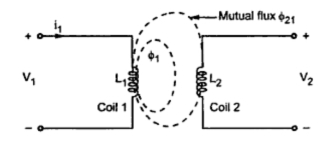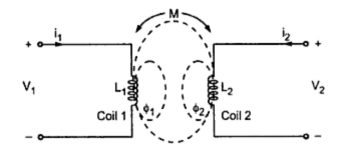### Introduction to Coupled Circuits

The two circuits are said to be coupled circuits if all or part of the electrical energy supplied to one circuit is transferred to the other circuit without having any electrical connection between them. Such coupled circuits are frequency used in the circuit analysis. The common examples of the coupled circuits are transformer, generator etc.
1. Magnetically Coupled Circuit
When the two circuits are placed very close to each other such that a magnetic flux produced by one circuit links with both the circuits, then the two circuits are said to be Magnetically Coupled Circuits. In this article, we will consider magnetically coupled circuits consisting coils or conductors. Such circuits are also called coupled inductors.
A wire of certain length, when twisted into coil becomes a basic inductor. If a current is made to pass through an inductor, an electromagnetic field is developed. A change in the magnitude of the current, changes the electromagnetic field and hence induces a voltage in coil according to Faraday's law of electromagnetic induction.
When two or more coils are placed very close to each other, then the current in one coil affects other coils by inducing voltage in them. Such coils are said to be mutually coupled coils. Such induced voltages in the coils are functions of the self inductances of the coils and mutual inductance between them. Let us study the concepts of the self inductance of a coil and mutual inductance.
1.1 Self Inductance
Consider a coil having N turns carrying current i as shown in the Fig. 1.Fig. 1  Self Inductance

Due to the current flow, the flux Φ is produced in the coil. The flux is measured in Wb (Weber). The flux produced by the coil links with the coil itself. Thus the total flux linkage of the coil will be (NΦ )Wb-turns. If the current flowing through the coil changes, the flux produced in the coil also changes and hence flux linkage also changes.
According to Faraday's law, due to the rate of change of flux linkages, there will be induced e.m.f. in the coil. This phenomenon is called self induction. The e.m.f. or voltage induced in the coil due to the change of its own flux linked with it, is called self induced e.m.f.
Key Point : the self induced e.m.f. lasts till the current in coil is changing. The direction of such induced e.m.f. is such that it opposes the cause producing it i.e. change in current.
Thus when current increases, the self induced e.m.f. reduces the current keeping it to its original value. And when current decreases, the self induced e.m.f increases it to maintain current to its original value.
Key Point : Any change in current through the coil is opposed by the coil.
The property of the coil which opposes any change in the current passing through it is called self inductance of coil or only inductance of coil.
According to Faraday's law of electromagnetic induction, self induced e.m.f. can be expressed as
V = -N (dΦ/dt)                                               ..........(1)
The negative sign indicates that the direction of the e.m.f. is opposing change in current due to which it exist
The flux Φ can be expressed as,
Φ = (Flux / Ampere) x Ampere = Φ/i  x  i
Hence,    Rate of change of flux = Φ/i  x  Rate of change of current
dΦ/dt = Φ/i  . di/dt                                           ............(2)
Putting value of dΦ/dt in equation (1), we can write,
V = -N(Φ/i . di/dt)
V = -(NΦ/i) di/dt                                                   .........(3)
The constant NΦ/i  is called coefficient of self inductance and denoted by L.
L = NΦ/i                                                                 .........(4)
Hence self induced e.m.f is given by
V= -L (di/dt)                                                       ................(5)
Thus the magnitude of the induced e.m.f. is given by
V = L (di/dt)                                                             ............(6)
Key point : The coefficient of self inductance is defined as the flux linkages per ampere current in it. Its unit is Henry (H).
1.2 Mutual Inductance
If the flux produced by one coil links with the other coil, placed sufficiently close to the first coil, then due to the change in the flux produced by first coil, there is induced e.m.f. in second coil. Such induced e.m.f. in the second coil is called mutually induced e.m.f.
Consider two coils which are placed very close to each other as shown in the Fig. 2.Fig. 2

Let coil 1 has N1 turns, while coil 2 has N2 turns. The current flowing through coil 1 is i1. Due to this current, the flux produced in coil 1 is Φ1. The part of this flux links with coil 2. This flux is called mutual flux.
It is denoted by Φ21 a sit is a part of flux Φ1 linking with coil 2. When current through coil 1 changes, the flux produced in coil 1 i.e. Φ1 changes. Thus flux associated with coil 2 i.e. Φ21 changes. So according to the Faraday's law, there will be induced e.m.f. in coil 2.
Key Point : Due to the changing current in coil 1, the e.m.f. is induced in coil 2. This phenomenon is called mutual induction and the induced e.m.f. is called mutually induced e.m.f.
According to Faraday's law, the magnitude of the induced e.m.f. is given by,
V2 = N2 (dΦ21/dt)                                              .................(7)
Now,        Φ21 = Φ21/i  x i
If the permeability of the surroundings is assumed constant then Φ21α    i1 and hence Φ21/i is constant.
...   Rate of change of Φ21 = Φ21/i  x  Rate of change of i1
...                 21/dt = Φ21/i1 . di1/dt                                           ...............(8)
Putting value of dΦ21/dt in equation (7), the magnitude of the induced e.m.f. can be written by as,
V2 = N2 21/i . di1/dt)
...                   V2  = (N2 Φ21/i1) di1/dt                                                 ...............(9)
Here the constant term (N2 Φ21/i1)is defined as coefficient of mutual inductance and it is denoted by M.
...                     M = N2 Φ21/ i1                                                           ...............(10)
Then equation (9) can be written as,
...                      V2  = M di1/dt                                                              .............(11)
Thus equation (10) indicates the magnitude of the e.m.f. induced in coil 2 as a function of change in current in coil 1.
Key Point : The coefficient of mutual inductance is the property by which e.m.f. gets induced in a coil because of change in current in other coil. It is also called mutual inductance and it is measured in Henry (H).
Let us consider two coils having self inductance L1 and L2 placed close to each other as shown on the Fig.3.Fig. 3

The two coils shown in above circuit are magnetically coupled but act as separate circuits. Due to current i1 ; the flux produced is Φ1. This flux links with coil 1 and coil 2 . Similarly due to current i2, the flux produced is  Φ2. This flux links with coil 2 as well as coil 1. So in each coil there will be self induced e.m.f. as well as mutually induced e.m.f.
Let M be the mutual inductance between the two coils. Then the magnitude of the self induced e.m.f. in coil 1 due to current i1 is L1 (di1/dt). The magnitude of the mutually induced e.m.f. in coil 1 due to current i2 in coil 2 is M di2/dt. Thus the magnitude of the total e.m.f. induced in coil 1 is given by,
V1  = L1 (di1/dt) + M(di2/dt)                                         ............(12)
Similarly, in coil 2, there will be self induced e.m.f. due to current i2 and mutually induced e.m.f. due to current i1 in coil 1. The n the magnitude of the total e.m.f. induced in coil 2 is given by,
V2  = L2 (di2/dt) + M (di1/dt                                          ................(13)
Key Point : In a pair of linear coupled circuits, a non-zero current in each coil produces mutually induced voltage in other coil and self induced voltage in same coil. The mutual inductance M is always positive but the mutually induced voltage may be either positive or negative depending upon the reference current directions and the physical construction of the coils.

Sponsored links :